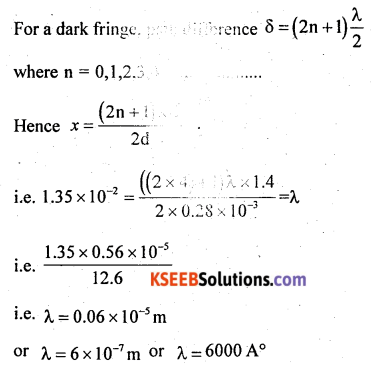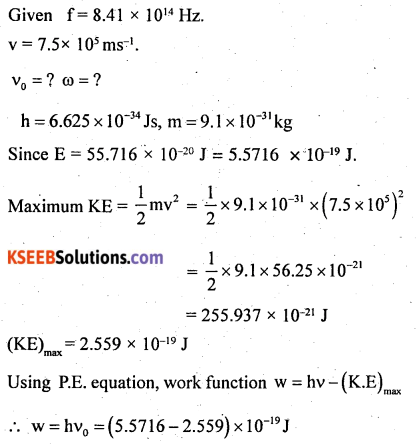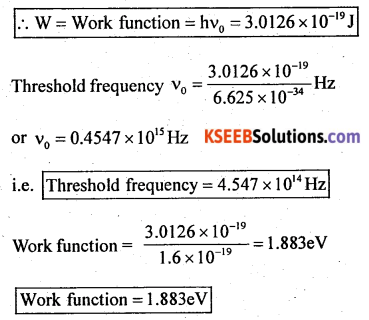# 2nd PUC Physics Previous Year Question Paper March 2018

Students can Download 2nd PUC Physics Previous Year Question Paper March 2018, Karnataka 2nd PUC Physics Model Question Papers with Answers helps you to revise the complete Karnataka State Board Syllabus and score more marks in your examinations.

## Karnataka 2nd PUC Physics Previous Year Question Paper March 2018

Time: 3 hrs 15 min
Max. Marks: 70

Instructions

• All parts are compulsory.
• Answer without relevant diagram/figure/circuit wherever necessary will not carry any marks.
• Numerical problems solved without writing the relevant formulae carry no marks.

Part – A

I. Answer all the following questions: (10 × 1 = 10)

Question 1.
What is an equipotential surface?
A surface on which electric potential remains the same at all points is called an equipotential surface.

Question 2.
Define ‘drift velocity’ of free electrons.
An average velocity with which an electron drift in a conductor in a direction opposite to the direction of applied electric field is called drift velocity.

Question 3.
Give an application of cyclotron.
The cyclotron is used to produce highly energetic charged particles or ions.Question 4.
State Faraday’s law of electromagnetic induction.
Whenever magnetic flux linked with the circuit changes, an emf is induced. The amount of emf induced is directly proportional to the time rate of change of magnetic flux linked with the circuit.

Question 5.
If the peak value of a.c. current is 4.24 A, what is its root mean square value?
Irms = $$\frac{I_{\text {peak }}}{\sqrt{2}}$$
= 0.707 × 4.24
= 2.9977 A
= 3.0 A

Question 6.
Mention one power loss in a transformer.
Copper loss, (flux linkage loss, eddy current loss, hysteresis loss).

Question 7.
Two lenses of power +1.5D and -0.5D are kept in contact on their principal axis. What is the effective power of the combination?
Effective power P = P1 + P2 = 1.5 – 0.5 = 1.0D

Question 8.
The decay of proton to neutron is possible only inside the nucleus. Why?
Unlike neutron, a proton is highly stable. Moreover, the rest mass energy of the proton is less than that of the neutron.Question 9.
What is ‘depletion region’ in a semiconductor diode?
A small region around the junction free from mobile charge carriers is called depletion region.

Question 10.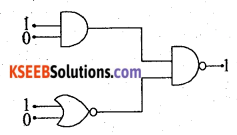What is the output of this combination?Output is ‘one’ or ‘high state’.

Part – B

II. Answer any five of the following questions: (5 × 2 = 10)

Question 11.
Mention any two factors on which the capacitance of a parallel plate capacitor depends.
The ratio of electric charge on the conductor to its electric potential is known as the electrical capacitance of the conductor.

Question 12.
State Kirchhoff’s laws of the electrical network.
Kirchoff’s law

1. Loop Law: The algebraic sum of the voltage drop across each component is equal to the supplied voltage by the source.
2. Junction Law: The net (incoming + outgoing) current at each junction should be zero.
In the case of the Wheatstone bridge, we know that the current through the galvanometer should be zero. i.e Ig = 0

Question 13.
Define magnetic ‘declination’ and ‘dip’ at a place.
North magnetic pole and south magnetic pole respectively.
A vertical plane containing the longitude circle and the axis of rotatioñ of the earth is called a geographic meridian.Question 14.
Write an expression for the magnetic potential energy of a magnetic dipole kept in a uniform magnetic field and explain the terms.
Magnetic potential energy
U = $$\overrightarrow{\mathrm{M}} \cdot \overrightarrow{\mathrm{B}}$$
m → magnetic moment of dipole
B → uniform magnetic field

Question 15.
Give any two applications of X-rays.
Broken bones. Today, x-rays are an integral part of contemporary hospitals and medical centres.
Radiation therapy. X-rays play an important role in the fight against cancer, with high energy radiation used to kill cancer cells and shrink tumours.

Question 16.
What is ‘myopia’? How to rectify it?
Nearsightedness or myopia is the inability of the eye to focus on distant objects. The nearsighted eye has no difficulty viewing nearby objects. But the ability to view distant objects requires that the light be refracted less.
Correction: The cure for the nearsighted eye is to equip it with a diverging lens. Since the light is focused in front of the retina, a diverging lens will serve to diverge light before it reaches the eye. This light will then be converted by the cornea and lens to produce an image on the retina.

Question 17.
Draw the diagram representing the schematic arrangement of Geiger-Marsden experimental set up for the alpha particle scattering.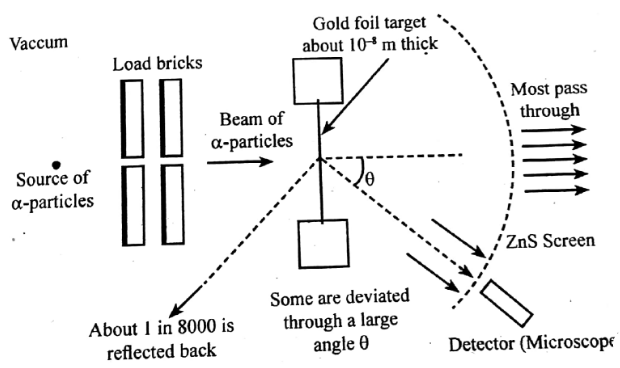Question 18.
Write any two characteristics of nuclear forces.

• It is attractive in nature but with a repulsive core.
• The range of a nuclear force is very short.
• The nuclear force is identical for all nucleons.
• At a distance of less than 0.7 Fermi, this force becomes repulsive.

Part – C

III. Answer any five of the following questions. (5 × 3 = 15)

Question 19.
Give three properties of electric charge.

• Charges are additive in nature
• A charge is a conserved quantity
• Quantization of chargeQuestion 20.
State Ampere’s circuital law and arrive at the expression for the magnetic field near a straight infinite current-carrying wire.
The integral of the products of the tangential component of the magnetic field and the element of length ¡n a closed loop is equal to µ0 times the total current passing through the surface.
$$\oint \overrightarrow{\mathrm{B}} \cdot d \vec{l}=\mu_{0} \mathrm{I}$$

Question 21.
What is hysteresis? Define the terms ‘coercivity’ and ‘retentivity’ of a ferromagnetic material.
Hysteresis: The phenomenon in which the magnetic induction ($$\vec{B}$$) lags behind with respect to the magnetizing field ($$\vec{H}$$) is called hysteresis.
It occurs when an external magnetic field is applied to a ferromagnet such as iron and the atomic dipoles align themselves with it. Even when the field is removed, part of the alignment will be retained: the material has become magnetized. Once magnetized, the magnet will stay magnetized indefinitely.

Retentivity: The property of the magnetic material to retain magnetism even in the absence of the magnetizing field is known as retentivity or remanence.

Coercivity: The magnetizing field (H) needed to demagnetize the magnetic material completely is known as its coercivity.

Question 22.
Arrive at Snell’s law of refraction, using Huygen’s principle for the refraction of a plane wave.
We know that when light travels from one transparent medium to another transparent medium its path changes. So the laws of refraction state that the angle of incidence is the angle between the incident ray and the normal and the angle of refraction is the angle between the refracted ray and the normal.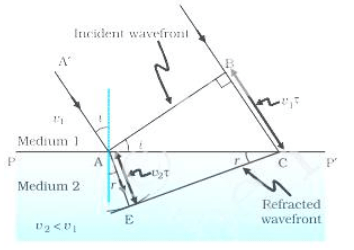The incident ray, reflected ray and the normal, to the interface of any two given mediums all lie in the same plane. We also know that the ratio of the sine of the angle of incidence and sine of the angle of refraction is constant.

Question 23.
Write Bohr’s postulates for the hydrogen atom model.

• The atom model provided the basic foundation for the development of quantum mechanics.
• The value of Rydberg’s constant R has arrived theoretically.
• The theory for the first time predicted the size of an atom in terms of absolute constants.
• This model made use of the principle of quantisation of angular momentum and energy states of hydrogen and hydrogenic atoms.

Question 24.
Derive an expression for the half-life of a radioactive nuclide.
Let’s find the relation between T½ and the disintegration constant λ. For this, let’s input the following values in the equation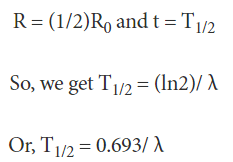Question 25.
Write any three distinctions between p-type and n-type semiconductor.
p-type Semiconductors

• Pure semiconductor ¡s doped with elements of III group (acceptors)
• Holes are majority charge carriers
• Energy levels of dopants are above the upper limit of the valence band.

n-type Semiconductors

• A pure semiconductor is doped with elements of V group (donors)
• Electrons are majority charge carriers.
• Energy levels of dopants are below the lower limit of the conduction band.Question 26.
Draw the block diagram df a generalised communication system.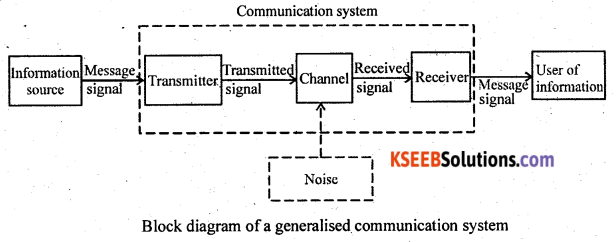Part – D

IV. Answer any two of the following questions: (2 × 5 = 10)

Question 27.
Define electric potential due to a point charge and derive at the expression for the electric potential at a point due to a point charge.
Consider the electric potential due to a point charge q, As we move from point A, at distance rA from the charge q, to point B, at distance rB from the charge q, the change in electric potential is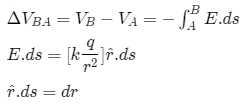Only the radial distance r determines the work is done or the potential. We can move through any angle we like and, as long as the radial distance remains constant, no work is done or there is no change in the electric potential.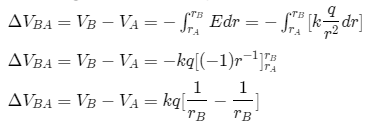This is the change in electric potential due to a point charge as we move from rA to rB.
We could ask about the change in electric potential energy as we move a charge q’ from radius rA to rB due to a point charge qAs with gravitational potential energy, it is more convenient and, therefore, useful to talk about the electric potential energy or the electric potential relative to some reference point. We will choose that reference point to be infinity. That is, rA = ∞
That means we can then write the electric potential at some radius r as V = kq $$\frac{1}{r}$$

Question 28.
Obtain an expression for the effective emf and the effective internal resistance of two cells connected in parallel such that the currents are flowing in the same direction.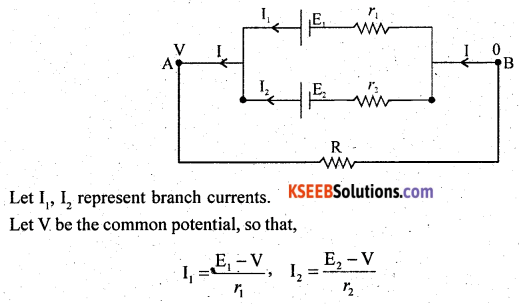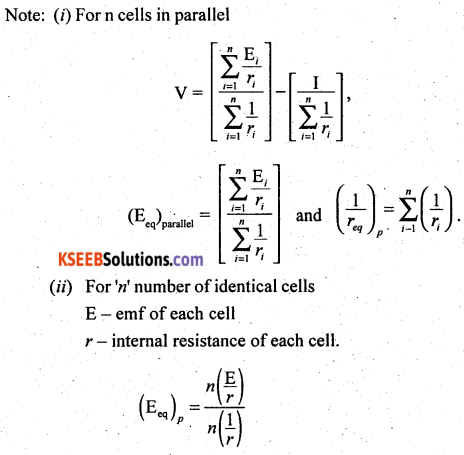Question 29.
Derive an expression for the magnetic field on the axis of a circular current loop, using Biot-Savart’s law.Let R be the radius of a current loop, carrying current I. Let R be a point on the axis of a conductor. Let dB be a magnetic field at P, due to a current element ‘idl’.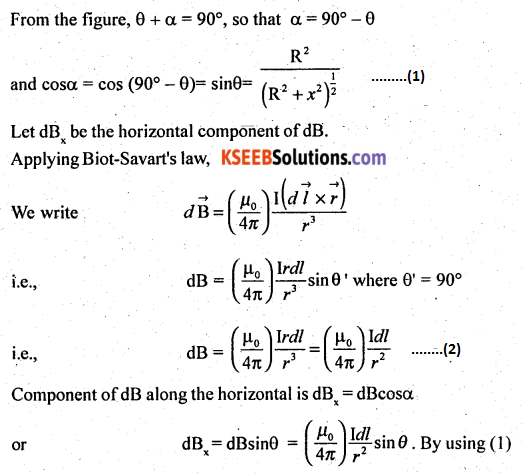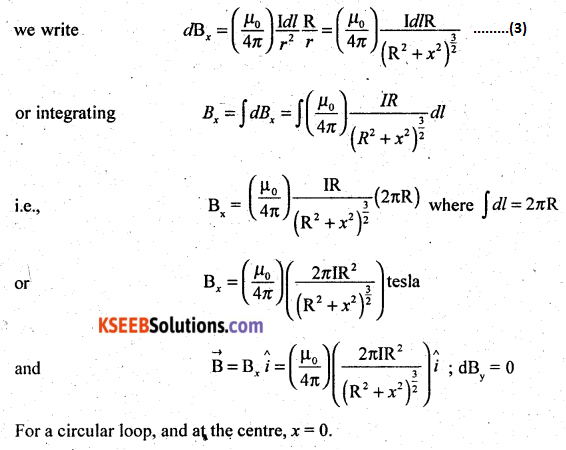V. Answer any two of the following questions: (2 × 5 = 10)

Question 30.
Arrive at an expression for the impedance of a series LCR circuit using phasor diagram method and hence write an expression for the current through the circuit.
Consider a resistance R, an inductor of self-inductance L and a capacitor of capacitance C connected in series across an AC source. The applied voltage is given by,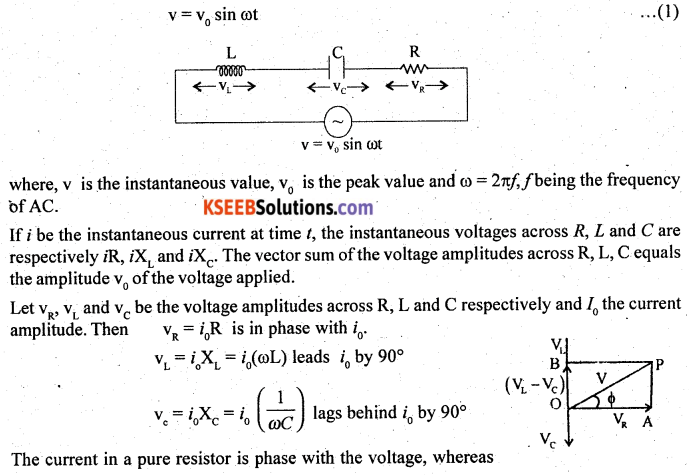Question 31.
Deduce the relation between n, u, v and R for refraction at a spherical surface, where the symbols have their usual meaning.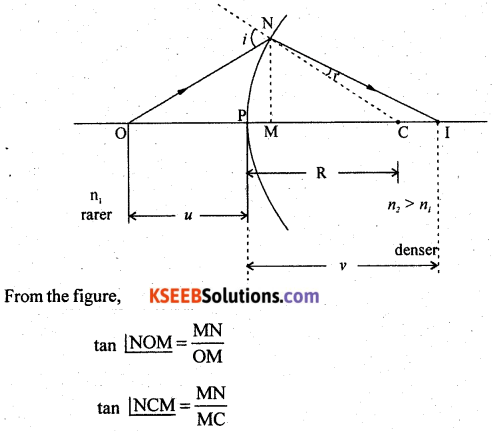Question 32.
What is a rectifier? With suitable circuit describe the action of a full-wave rectifier by drawing input and output waveforms.
Full-wave rectification: The process in which both the halves of a.c. are rectified to obtain a pulsating direct current is known as full-wave rectification.
A transformer with a centre tap is used for full-wave rectification. The input contains ac. A minimum of two diodes are used at the secondary of the transformer. A load resistor is connected between the junction of the N-type semiconductor and the centre tap.
During the first half cycle, diode D1 will be forward biased and D2 will be reverse biased so that the current flows from B to centre tap.During the second half cycle, diode, D2 will be forward biased while D1 will be reverse biased. The current once again takes the same direction from B to the centre tap. The I/P and the O/P waveforms are shown in fig.
The average DC is $$2\left(\frac{V_{p}}{\pi}\right)$$VI. Answer any three of the following questions: (3 × 5 = 15)

Question 33.
Three charges each equal to +4nC are placed at the three corners of a square of side 2 cm. Find the electric field at the fourth corner.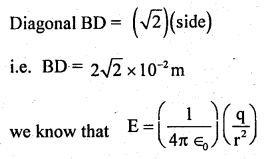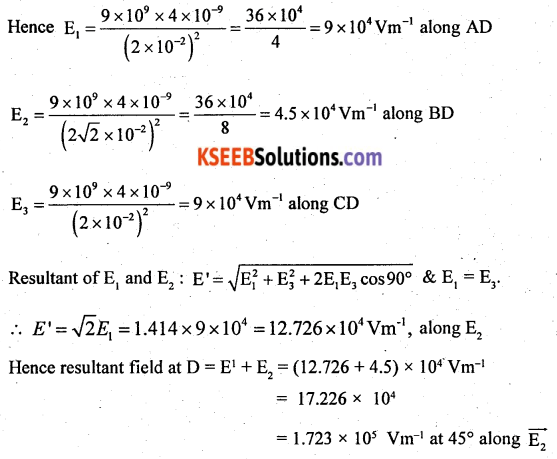Question 34.
100 mg mass of nichrome metal is drawn into a wire of area of cross-section 0.05 mm2. Calculate the resistance of this wire. Given the density of nichrome 8.4 × 103 kgm-3 and resistivity of the material as 1.2 × 10-6 Ωm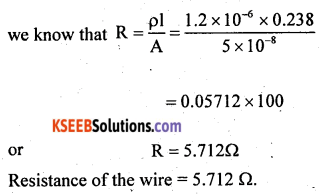Question 35.
A circular coil of radius 10 cm and 25 turns is rotated about its vertical diameter with an angular speed of 40 rad s-1, in a uniform horizontal magnetic field of magnitude 5 × 10-2 T. Calculate the maximum emf induced in the coil. Also, find the maximum current in the coil if the resistance of the coil is 15 Ω.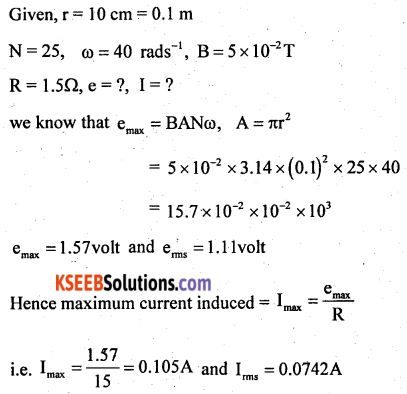Question 36.
In Young’s double-slit experiment the slits are separated by 0.28 mm and the screen is placed at a distance of 1.4 m away from the slits. The distance between the central bright fringe and the fifth dark fringe is measured to be 1.35 cm. Calculate the wavelength of the light used. Also find the fringe width if the screen is moved towards the slits, for the same experimental setup.